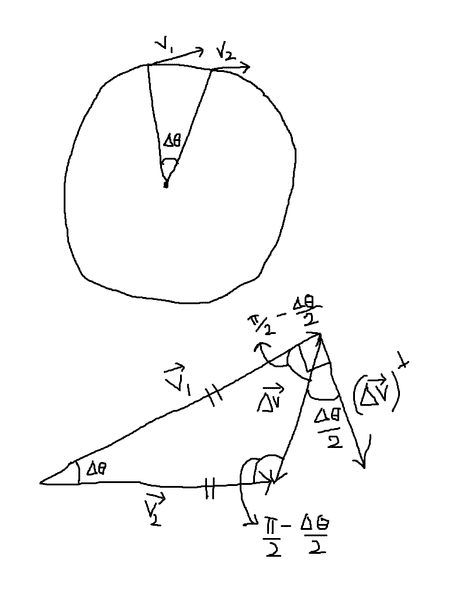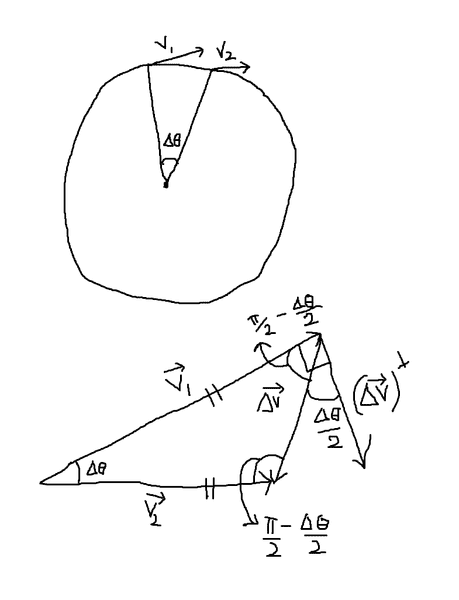# Centripetal acceleration derivation

• Andreas C

#### Andreas C

Ok, so I'm a bit confused by the derivation of a=v^2/r in Feynman's "Six Not-So-Easy Pieces".

In pages 17-18, it is stated that "The other component of acceleration, at right angles to the curve, is easy to calculate, using Figures 1-7 and 1-8. In the short time Δt let the change in angle between v1 and v2 be the small angle Δθ. If the magnitude of the velocity is called v, then of course Δv ┴ = vΔθ and the acceleration a will be a┴ = v(Δθ/Δt).".

1) What velocity does he refer to by v?
2) How can he multiply Δθ (an angle) by v (a magnitude of a velocity)?
3) (Probably the same as 2) How can he divide Δθ by Δt?

I haven't read the rest of the book, so maybe that's where the answer is? I don't know, it seems very weird.

1) What velocity does he refer to by v?
the net resultant velocity

2) How can he multiply Δθ (an angle) by v (a magnitude of a velocity)?
the same you multiply mass by acceleration to get force
3) (Probably the same as 2) How can he divide Δθ by Δt?
same way you divide distance by time to get speed

the net resultant velocity

the same you multiply mass by acceleration to get force

same way you divide distance by time to get speed

Yeah, but he multiplies speed by an angle, and he gets velocity! How? And then he divides an angle with time, and multiplies it by speed, and gets acceleration!

An angle is a ratio of two length and thus itself dimensionless, namely arclength of the section of a circle divided by the radius of the circle. So any quantity multiplied by an angle is another quantity with the same dimension as the one you multiplied with the angle.

Ah, I think I am beginning to understand! So this angle would be measured in radians, not degrees, right? But what does this achieve though? What does multiplying an angle by speed achieve in this context?

When you rotate the radius by an angle θ, the arc described by the tip of the radius is s=Rθ.
Here the radius is v and the angle is very small so the cord and the arc are assumed the same thing.$$\triangle{v(\bot)}=\sqrt{v1^{2}+v2^{2}-2\cdot{v1}\cdot{v2}\cdot{cos(\triangle\theta)}}\cdot{cos(\triangle\theta/2)}$$
=$$\sqrt{2v^{2}-2v^{2}\cdot{cos(\triangle\theta)}}\sqrt{\frac{1+cos(\triangle\theta)}{2}}$$
=$$v\sqrt{1-{cos(\triangle\theta)}^{2}}$$
=$$v\cdot{sin(\triangle\theta)}$$
=$$v\triangle\theta$$

•Andreas C
Oh thanks, I get it now!

speaking as adults when kids have gone to bed... :)

Let ##\boldsymbol r=\boldsymbol r(s)## be the space curve parameterized by arc-length parameter ##s##. And let ##\boldsymbol{T}(s),\boldsymbol{N}(s),\boldsymbol{B}(s)## be the Frenet frame. By ##\kappa(s),\tau(s)## denote the curvature and torsion respectively. https://en.wikipedia.org/wiki/Frenet–Serret_formulas
Assume that a point ##A## moves on the curve and its law of motion is ##s=s(t)##.

Theorem. The acceleration of the point ##A## is given by the formula ##\boldsymbol a_A=\ddot s\boldsymbol T+\dot s^2\kappa\boldsymbol{N}.##

(It follows immediately from the Frenet-Serret formulas by differentiating the following equality ##\boldsymbol v_A=\dot r=\dot s\boldsymbol T.##$$\triangle{v(\bot)}=\sqrt{v1^{2}+v2^{2}-2\cdot{v1}\cdot{v2}\cdot{cos(\triangle\theta)}}\cdot{cos(\triangle\theta/2)}$$
=$$\sqrt{2v^{2}-2v^{2}\cdot{cos(\triangle\theta)}}\sqrt{\frac{1+cos(\triangle\theta)}{2}}$$
=$$v\sqrt{1-{cos(\triangle\theta)}^{2}}$$
=$$v\cdot{sin(\triangle\theta)}$$
=$$v\triangle\theta$$
Note that it is a rule on the Forums to give hints and not full solutions.

speaking as adults when kids have gone to bed... :)

Let ##\boldsymbol r=\boldsymbol r(s)## be the space curve parameterized by arc-length parameter ##s##. And let ##\boldsymbol{T}(s),\boldsymbol{N}(s),\boldsymbol{B}(s)## be the Frenet frame. By ##\kappa(s),\tau(s)## denote the curvature and torsion respectively. https://en.wikipedia.org/wiki/Frenet–Serret_formulas
Assume that a point ##A## moves on the curve and its law of motion is ##s=s(t)##.

Theorem. The acceleration of the point ##A## is given by the formula ##\boldsymbol a_A=\ddot s\boldsymbol T+\dot s^2\kappa\boldsymbol{N}.##

(It follows immediately from the Frenet-Serret formulas by differentiating the following equality ##\boldsymbol v_A=\dot r=\dot s\boldsymbol T.##

Uh... I have no idea what you just said, but thanks I guess...

Note that it is a rule on the Forums to give hints and not full solutions.

It is? Why?

Uh... I have no idea what you just said, but thanks I guess...
I said that the formula you are trying to derive is a special case of the general fact which is valid for any spatial curve not only for circle. And it takes one line to deduce that general formula

Last edited:
I said that the formula you are trying to derive is a special case of the general fact which is valid for any spatial curve not only for circle. And it takes one line to deduce that general formula

Ah ok. But it only takes one line given that you know the rest of the theory behind it.